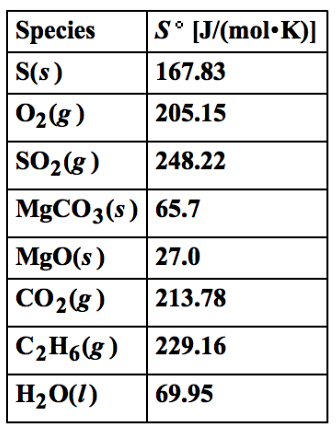# Problem: Using the data in the table, calculate the standard entropy changes (J/mol•K) for the following reactions at 25 °C.S(s) + O2(g) → SO2(g)MgCO3(s) → MgO(s) + CO2(g)2 C2H6(g) + 7O2(g) → 4 CO2(g) + 6 H2O(l)

###### FREE Expert Solution
79% (204 ratings)
###### FREE Expert Solution
79% (204 ratings)###### Problem Details

Using the data in the table, calculate the standard entropy changes (J/mol•K) for the following reactions at 25 °C.

S(s) + O2(g) → SO2(g)

MgCO3(s) → MgO(s) + CO2(g)

2 C2H6(g) + 7O2(g) → 4 CO2(g) + 6 H2O(l)What scientific concept do you need to know in order to solve this problem?

Our tutors have indicated that to solve this problem you will need to apply the Entropy concept. You can view video lessons to learn Entropy. Or if you need more Entropy practice, you can also practice Entropy practice problems.

What is the difficulty of this problem?

Our tutors rated the difficulty ofUsing the data in the table, calculate the standard entropy ...as medium difficulty.

How long does this problem take to solve?

Our expert Chemistry tutor, Dasha took 6 minutes and 57 seconds to solve this problem. You can follow their steps in the video explanation above.

What professor is this problem relevant for?

Based on our data, we think this problem is relevant for Professor Tucker's class at CSU.